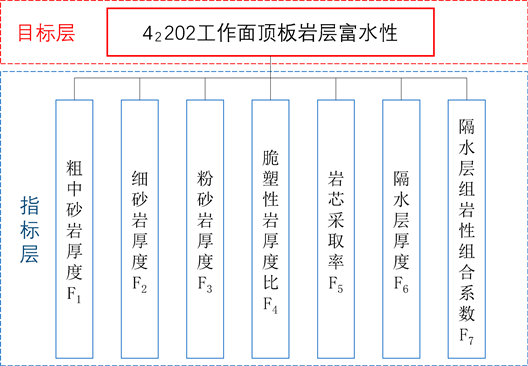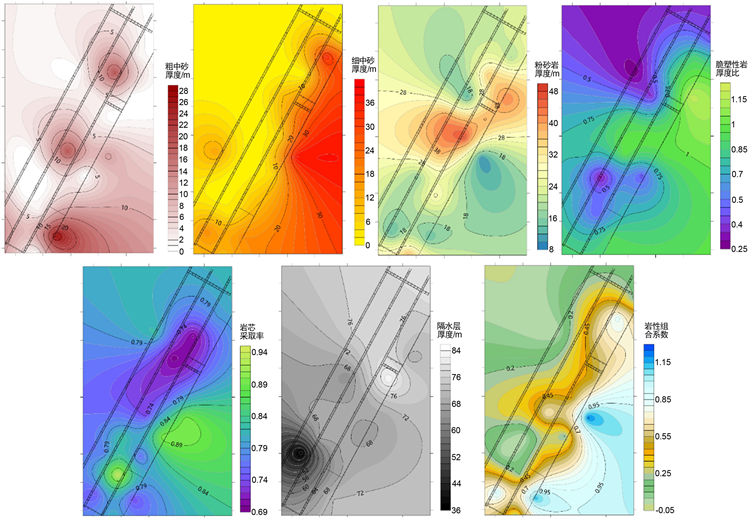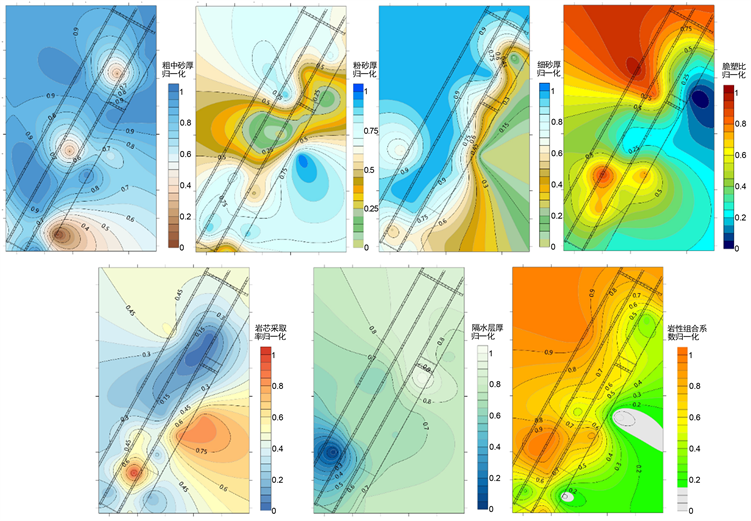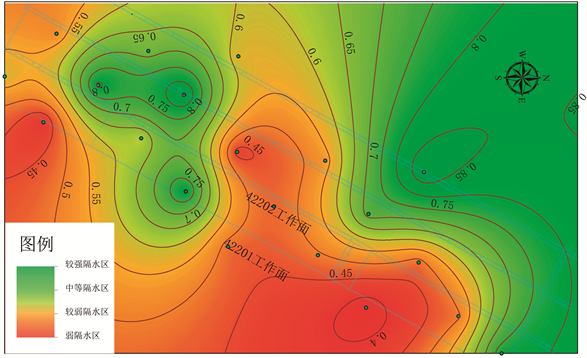﻿ 基于FDAHP与独立性权系数综合确权的煤层顶板隔水性能评价

基于FDAHP与独立性权系数综合确权的煤层顶板隔水性能评价Evaluation of Coal Seam Roof Water-Resistance Performance Based on FDAHP and Independent Weight Coefficient Method

Abstract: Traditional multi-source information fusion methods with single subjective or objective weighting are often used when evaluating the water-resistance performance of coal seam roof. To avoid these inadequacies, 7 major controlling factors including the coarse and medium sandstone thickness, fine sandstone thickness, siltstone thickness, brittle-plastic rock thickness ratio, core monitor rate, waterproof layer thickness and lithology combination index of water-resisting layer group were selected based on the fully consideration of various factors affecting the No. 4 coal roof water-resistance. This paper calculated the subjective and objective weights of each main controlling factor respectively by the FDAHP and independent weight coefficient methods, and obtained the comprehensive weight by the normalization; using Arcgis to establish a partition map of the water-resistance performance of coal seam roof. The results show that the water resistance in the northwest direction of the area is strong, obvious fitting effect of the inclination direction of the mining face, the water-resistance performance of coal seam roof in 42202 working face is between medium strength and weak, which is better than that of the 42201 working face.

1. 引言

2. 研究区概况

3. 主控因素的确定及量化Figure 1. Water-rich hierarchical structure model of roof rock formation

3.1. 含水层结构因素

42202工作面煤层顶板的主要储水层为砂岩含水层，在其他因素一致的情况下，储水能力与含水层厚度呈正比，即含水层越厚，煤层顶板隔水性能越弱；42202工作面顶板煤系砂岩主要含水岩性为粗砂岩和中砂岩，细砂岩和粉砂岩次之，统计不同岩性岩层的厚度数据，分别建立粗、中砂岩，细砂岩和粉砂岩厚度的专题图，图2

3.2. 岩层完整性因素

3.3. 隔水层结构因素Figure 2. Thematic map of each main control factor

4. 主、客观权重的确定

4.1. 基于FDAHP的主观赋权

4.1.1. 建立比较判断矩阵Table 1. Evaluation value of the importance of each main control factor to the water richness of coal roof

$A=\left[\begin{array}{ccccccc}1& {a}_{12}& {a}_{13}& {a}_{14}& {a}_{15}& {a}_{16}& {a}_{17}\\ 1/{a}_{12}& 1& {a}_{23}& {a}_{24}& {a}_{25}& {a}_{26}& {a}_{27}\\ 1/{a}_{13}& 1/{a}_{23}& 1& {a}_{34}& {a}_{35}& {a}_{36}& {a}_{37}\\ 1/{a}_{14}& 1/{a}_{24}& 1/{a}_{34}& 1& {a}_{45}& {a}_{46}& {a}_{47}\\ 1/{a}_{15}& 1/{a}_{25}& 1/{a}_{35}& 1/{a}_{45}& 1& {a}_{56}& {a}_{57}\\ 1/{a}_{16}& 1/{a}_{26}& 1/{a}_{36}& 1/{a}_{46}& 1/{a}_{56}& 1& {a}_{67}\\ 1/{a}_{17}& 1/{a}_{27}& 1/{a}_{37}& 1/{a}_{47}& 1/{a}_{57}& 1/{a}_{67}& 1\end{array}\right]$

${A}_{P2}=\left[\begin{array}{ccccccc}1.000& 2.000& 2.000& 1.333& 1.333& 1.000& 1.143\\ 0.500& 1.000& 1.000& 0.667& 0.667& 0.500& 0.571\\ 0.500& 1.000& 1.000& 0.667& 0.667& 0.500& 0.571\\ 0.750& 1.500& 1.500& 1.000& 1.000& 0.750& 0.857\\ 0.750& 1.500& 1.500& 1.000& 1.000& 0.750& 0.857\\ 1.000& 2.000& 2.000& 1.333& 1.333& 1.000& 1.143\\ 0.875& 1.750& 1.750& 1.167& 1.167& 0.875& 1.000\end{array}\right]$

${A}_{P3}=\left[\begin{array}{ccccccc}1.000& 1.333& 1.600& 1.600& 1.600& 1.333& 1.143\\ 0.750& 1.000& 1.200& 1.200& 1.200& 1.000& 0.857\\ 0.625& 0.833& 1.000& 1.000& 1.000& 0.833& 0.714\\ 0.625& 0.833& 1.000& 1.000& 1.000& 0.833& 0.714\\ 0.625& 0.833& 1.000& 1.000& 1.000& 0.833& 0.714\\ 0.750& 1.000& 1.200& 1.200& 1.200& 1.000& 0.857\\ 0.875& 1.167& 1.400& 1.400& 1.400& 1.167& 1.000\end{array}\right]$

${A}_{P4}=\left[\begin{array}{ccccccc}1.000& 2.000& 2.000& 1.333& 1.333& 1.000& 2.000\\ 0.500& 1.000& 1.000& 0.667& 0.667& 0.500& 1.000\\ 0.500& 1.000& 1.000& 0.667& 0.667& 0.500& 1.000\\ 0.750& 1.500& 1.500& 1.000& 1.000& 0.750& 1.500\\ 0.750& 1.500& 1.500& 1.000& 1.000& 0.750& 1.500\\ 1.000& 2.000& 2.000& 1.333& 1.333& 1.000& 2.000\\ 0.500& 1.000& 1.000& 0.667& 0.667& 0.500& 1.000\end{array}\right]$

${A}_{P5}=\left[\begin{array}{ccccccc}1.000& 1.500& 1.500& 1.000& 1.000& 1.000& 1.200\\ 0.667& 1.000& 1.000& 0.667& 0.667& 0.667& 0.800\\ 0.667& 1.000& 1.000& 0.667& 0.667& 0.667& 0.800\\ 1.000& 1.500& 1.500& 1.000& 1.000& 1.000& 1.200\\ 1.000& 1.500& 1.500& 1.000& 1.000& 1.000& 1.200\\ 1.000& 1.500& 1.500& 1.000& 1.000& 1.000& 1.200\\ 0.833& 1.125& 1.125& 0.833& 0.833& 0.833& 1.000\end{array}\right]$

${A}_{P6}=\left[\begin{array}{ccccccc}1.000& 1.400& 1.400& 1.167& 1.400& 1.167& 1.400\\ 0.714& 1.000& 1.000& 0.833& 1.000& 0.833& 1.000\\ 0.714& 1.000& 1.000& 0.833& 1.000& 0.833& 1.000\\ 0.857& 1.200& 1.200& 1.000& 1.200& 1.000& 1.200\\ 0.714& 1.000& 1.000& 0.833& 1.000& 0.833& 1.000\\ 0.857& 1.200& 1.200& 1.000& 1.200& 1.000& 1.200\\ 0.714& 1.000& 1.000& 0.833& 1.000& 0.833& 1.000\end{array}\right]$

${A}_{P7}=\left[\begin{array}{ccccccc}1.000& 1.000& 1.000& 0.857& 1.200& 0.750& 0.857\\ 1.000& 1.000& 1.000& 0.857& 1.200& 0.750& 0.857\\ 1.000& 1.000& 1.000& 0.857& 1.200& 0.750& 0.857\\ 1.167& 1.167& 1.167& 1.000& 1.400& 0.875& 1.000\\ 0.833& 0.833& 0.833& 0.714& 1.000& 0.625& 0.714\\ 1.333& 1.333& 1.333& 1.143& 1.600& 1.000& 1.143\\ 1.167& 1.167& 1.167& 1.000& 1.400& 0.875& 1.000\end{array}\right]$

${A}_{P8}=\left[\begin{array}{ccccccc}1.000& 1.000& 1.000& 0.667& 0.800& 1.000& 0.800\\ 1.000& 1.000& 1.000& 0.667& 0.800& 1.000& 0.800\\ 1.000& 1.000& 1.000& 0.667& 0.800& 1.000& 0.800\\ 1.500& 1.500& 1.500& 1.000& 1.200& 1.500& 1.200\\ 1.125& 1.125& 1.125& 0.833& 1.000& 1.125& 1.000\\ 1.000& 1.000& 1.000& 0.667& 0.800& 1.000& 0.800\\ 1.125& 1.125& 1.125& 0.833& 1.000& 1.125& 1.000\end{array}\right]$

4.1.2. 建立群体模糊判断矩阵

$B=\left({b}_{ij}\right)$ (1)

$\left\{\begin{array}{l}{\alpha }_{ij}=\mathrm{min}\left({a}_{ijk}\right)\\ {\beta }_{ij}={\left({\prod }_{k=1}^{m}{a}_{ijk}\right)}^{\frac{1}{m}}\\ {\gamma }_{ij}=\mathrm{max}\left({a}_{ijk}\right)\end{array}\text{\hspace{0.17em}}\text{\hspace{0.17em}}\text{\hspace{0.17em}}\left(k=1,\cdots ,m\right)$ (2)4.1.3. 确定群体模糊权重向量

$\left\{\begin{array}{l}{r}_{i}=\sqrt[n]{\left({a}_{i1}\otimes {a}_{i2}\otimes \cdot \cdot \cdot \otimes {a}_{in}\right)}\\ {w}_{i}={r}_{i}\otimes {\left({r}_{1}\oplus {r}_{2}\oplus \cdot \cdot \cdot \oplus {r}_{n}\right)}^{-1}\\ {w}_{i}=\left({w}_{i}^{L},{w}_{i}^{M},{w}_{i}^{U}\right)\end{array}$ (3)

$\left\{\begin{array}{l}a\otimes b=\left({a}_{1}×{b}_{1},{a}_{2}×{b}_{2},{a}_{3}×{b}_{3}\right)\\ a\oplus b=\left({a}_{1}+{b}_{1},{a}_{2}+{b}_{2},{a}_{3}+{b}_{3}\right)\\ {b}^{-1}=\left(\frac{1}{{b}_{3}},\frac{1}{{b}_{2}},\frac{1}{{b}_{1}}\right)\end{array}$ (4)

$\left\{\begin{array}{l}{w}_{1}=\left(0.065,0.122,0.226\right)\hfill \\ {w}_{2}=\left(0.096,0.168,0.284\right)\hfill \\ {w}_{3}=\left(0.102,0.177,0.306\right)\hfill \\ {w}_{4}=\left(0.075,0.139,0.280\right)\hfill \\ {w}_{5}=\left(0.075,0.142,0.254\right)\hfill \\ {w}_{6}=\left(0.063,0.120,0.220\right)\hfill \\ {w}_{7}=\left(0.070,0.133,0.263\right)\hfill \end{array}$

4.1.4. 权重决策分析

${W}_{i}=\frac{{\left({w}_{i}^{L}{w}_{i}^{M}{w}_{i}^{U}\right)}^{\frac{1}{3}}}{{\sum }_{i}{\left({w}_{i}^{L}{w}_{i}^{M}{w}_{i}^{U}\right)}^{\frac{1}{3}}},\text{\hspace{0.17em}}i=\left(1,2,\cdots ,n\right)$ (5)

4.2. 基于独立性权系数法的客观赋值

$\left\{\begin{array}{l}{R}_{i}=\frac{{\sum }^{\text{​}}\left({X}_{i}-\stackrel{¯}{X}\right)\left(\stackrel{˜}{X}-\stackrel{¯}{X}\right)}{\sqrt{{\sum }^{\text{​}}{\left({X}_{i}-\stackrel{¯}{X}\right)}^{2}{\left(\stackrel{˜}{X}-\stackrel{¯}{X}\right)}^{2}}}\\ {D}_{i}=\frac{\frac{1}{{R}_{i}}}{{\sum }_{i=1}^{m}\frac{1}{{R}_{i}}}\end{array}\text{\hspace{0.17em}}\text{\hspace{0.17em}}\text{\hspace{0.17em}}i=\left(1,2,\cdots ,m\right)$ (6)Table 2. The objective weight of each main control factor

5. 数学模型的建立

5.1. 数据归一化处理

${x}_{i}^{\ast }=\frac{{x}_{i}-{x}_{\mathrm{min}}}{{x}_{\mathrm{max}}-{x}_{\mathrm{min}}}$ (7a)

${x}_{i}^{\ast }=\frac{{x}_{i}-{x}_{\mathrm{max}}}{{x}_{\mathrm{m}\text{ax}}-{x}_{\mathrm{min}}}$ (7b)

xi——评价指标数据归一化前的值； ${x}_{i}^{\ast }$ ——评价指标数据归一化后的值；xmin——某评价指标数据中的最小值；xmax——某评价指标数据中的最大值。Figure 3. The normalized thematic map of each main control factor

5.2. 隔水性能指数数学模型的建立

${C}_{i}=\frac{{W}_{i}\cdot {D}_{i}}{{\sum }_{i=1}^{n}{W}_{i}\cdot {D}_{i}}$ (8)

$\begin{array}{c}{C}_{t}={\sum }_{i=1}^{n}{C}_{i}{F}_{i}\left(x,y\right)\\ =0.107{F}_{1}\left(x,y\right)+0.187{F}_{2}\left(x,y\right)+0.194{F}_{3}\left(x,y\right)+0.140{F}_{4}\left(x,y\right)\\ \text{\hspace{0.17em}}\text{ }+0.141{F}_{5}\left(x,y\right)+0.100{F}_{6}\left(x,y\right)+0.131{F}_{7}\left(x,y\right)\end{array}$ (9)

5.3. 工作面顶板隔水性能分区与评价Table 3. The water-impermeability partition table of the roofFigure 4. The partition diagram of the roof water-proof performance

6. 结论

1) 区内西北向的隔水性能较强，与采掘工作面倾斜方向拟合效果明显，42202工作面煤层底板岩层阻隔水性能在中等强度到弱之间，优于旁边的42201工作面。

2) 在缺少足够的钻孔单位涌水量资料情况下，脆塑性岩厚度比，隔水层岩性组合系数，岩芯采取率等可作为评价工作面顶板隔水性能的主控指标

3) 采用FDAHP与独立行权系数法综合确权的方法，可应用于该区工作面顶板隔水性能的评价，为矿井水害的防治提供一定的理论基础。

 张伟杰, 李术才, 魏久传, 等. 岩溶泉域煤矿奥灰顶部相对隔水性及水文地质特征研究[J]. 岩石力学与工程学报, 2014, 33(2): 349-357.

 王洋. 煤矿充水含水层富水规律与分区评价及疏降水量动态预测[D]: [博士学位论文]. 北京: 中国矿业大学, 2017.

 石守桥, 魏久传, 尹会永, 等. 济三煤矿煤层顶板砂岩含水层富水性预测[J]. 煤田地质与勘探, 2017, 45(5): 100-104.

 武强, 樊振丽, 刘守强, 等. 基于GIS的信息融合型含水层富水性评价方法——富水性指数法[J]. 煤炭学报, 2011, 36(7): 1124-1128.

 陈康, 薛国强, 陈卫营, 等. 一种煤层顶板富水性快速评价方法[J]. 地球物理学进展, 2017, 32(4): 1752-1757.

 贾鹏宙. 煤矿床含水层富水性定量评价方法研究[J]. 煤炭科学技术, 2017, 45(2): 168-172.

 李刚, 李建平, 孙晓蕾, 等. 主客观权重的组合方式及其合理性研究[J]. 管理评论, 2017, 29(12): 17-26+61.

 蔡海兵, 程桦. 基于FDAHP理论的深部岩体分级方法[J]. 水文地质工程地质, 2012, 39(6): 43-49.

 邱梅, 施龙青, 滕超, 等. 基于灰色关联-FDAHP法与物探成果相结合的奥灰富水性评价[J]. 岩石力学与工程学报, 2016, 35(S1): 3203-3213.

Top